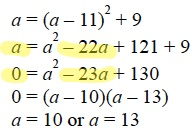PWN the SAT Parabolas drill explanation p. 325 #10: The final way to solve: If we are seeking x=y, since the point is (a,a), why can you set f(x) = 0? You start out with the original equation in vertex form, making y=a and x=a, but halfway through you change to y=0 (while x is still = a). How can we be solving the equation when we no longer have a for both x and y?

For everyone else’s context, here’s the problem:Now, be careful! I am not changing to y = 0 in that algebraic solution in the back of the book; I am subtracting a from both sides! Note how the a term on the right changes from –22a to –23a.